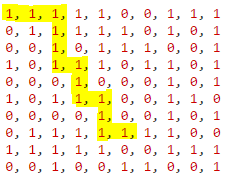# Shortest path in a Maze | Lee algorithm

Given a maze in the form of the binary rectangular matrix, find length of the shortest path in a maze from given source to given destination.

The path can only be constructed out of cells having value 1 and at any given moment, we can only move one step in one of the four directions. The Valid moves are:

Go Up: (x, y) –> (x – 1, y)
Go Left: (x, y) –> (x, y – 1)
Go Down: (x, y) –> (x + 1, y)
Go Right: (x, y) –> (x, y + 1)

For example, consider below binary matrix. If source = (0, 0) and destination = (7, 5), the shortest path from source to destination has length 12.We have already discussed a backtracking solution in previous post. The time complexity of above backtracking solution will be higher since all paths need to be traveled. However, since it is an shortest path problem, BFS would be an ideal choice.

The Lee algorithm is one possible solution for maze routing problems based on Breadth-first search. It always gives an optimal solution, if one exists, but is slow and requires considerable memory. Below is the complete algorithm –

1. Create an empty queue and enqueue source cell having distance 0 from source (itself) and mark it as visited

2. do till queue is not empty
• Pop front node from the queue

• If the popped node is destination node, then return its distance

• else for each of 4 adjacent cells of current cell, we enqueue each valid cell into the queue with +1 distance and mark them as visited

3. If all the nodes in the queue is processed and destination is not reached, then return false

Note that in BFS, all cells having shortest path as 1 are visited first, followed by their adjacent cells having shortest path as 1 + 1 = 2 and so on.. so if we reach any node in BFS, its shortest path = shortest path of parent + 1. So, the first occurrence of the destination cell gives us the result and we can stop our search there. It is not possible that the shortest path exists from some other cell for which we haven’t reached the given node yet. If any such path was possible, we would have already explored it.

## C++

Output:

The shortest path from source to destination has length 12

## Java

Output:

The shortest path from source to destination has length 12

Time complexity of above solution is O(MN).(7 votes, average: 4.43 out of 5)Loading...

Please use our online compiler to post code in comments. To contribute, get in touch with us.
Like us? Please spread the word and help us grow. Happy coding 🙂Subscribe
Notify ofGuest

this is one of the finest blog I have ever read to understand BFS and DFS. thanks a ton.Guest

Thanks a lottGuest

Awesome!!Guest

How come moving diagonally is not allowed?